# 零基础微积分入门基本教程（一）

w568w 本文已被读

（收到了1800次阅读，有点小开心呐~~~~）

# 参考书籍

1. 《高等数学（第六版）》上册
同济大学数学系 编
高等教育出版社 出版
2. 《7天搞定微积分》
石山平 大上丈彦 著 李巧丽 译
南海出版公司 出版

1. 至少初中水平的数学应用能力
2. 至少小学水平的阅读能力
3. 耐心和对数学的热情
4. 以上三条是扯淡
5. 这条也是

# 一.导数

导数是微分学的主要内容。翻回去看看百度百科对导数的解释：

## 1.1 First Blood —— 斜率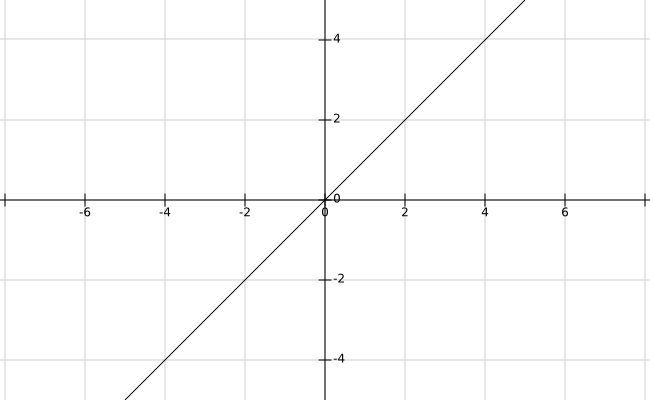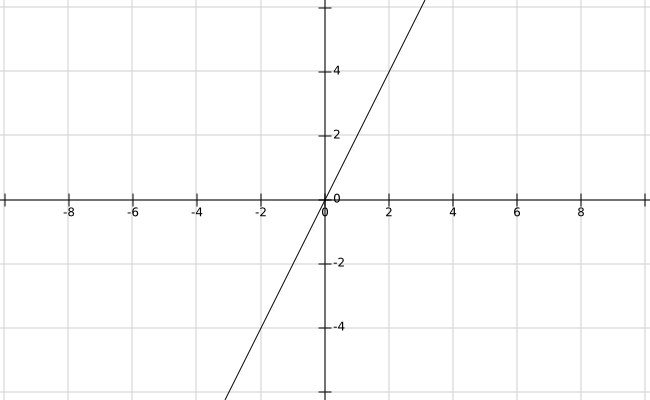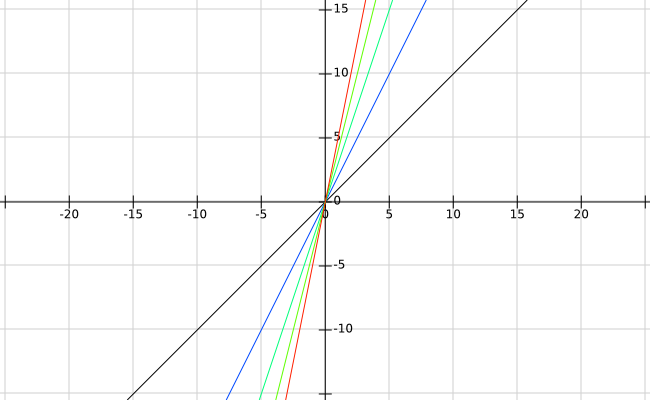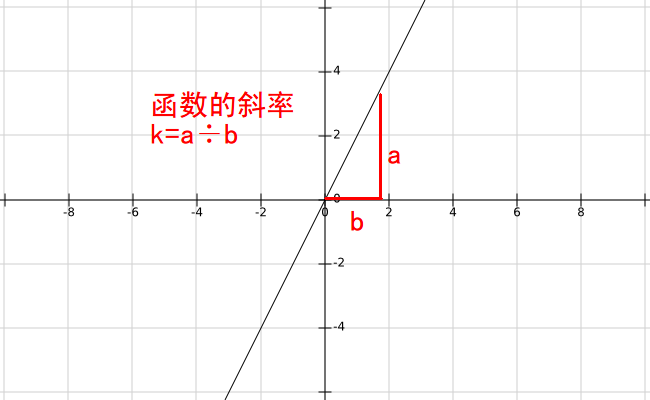### 1.1.1 [选读]斜率的严格定义

（对于初三及以上水平的读者，你们可以有选择性地阅读这些标有”[选读]“文字的部分，因为这些部分可以加深你对一些知识的理解。如果你不想看， 跳过也没关系

slope，又称“角系数”，是一条直线对于横坐标轴正向夹角的正切，反映直线对水平面的倾斜度。## 1.2 点的斜率？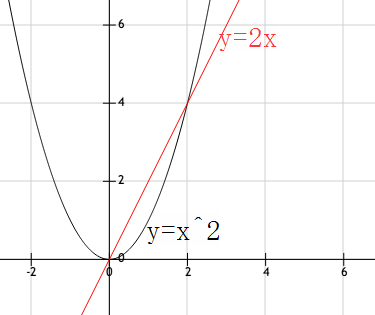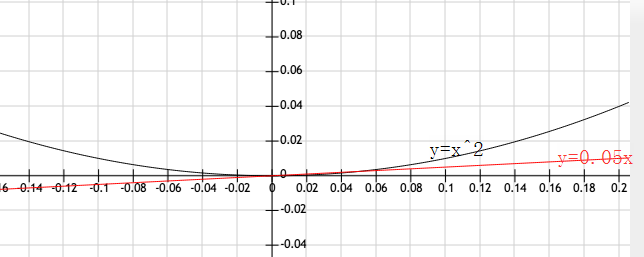PS: 从这里我开始尝试使用 GeoGebra 来作图，网页版的画图实在是太!蛋!疼!了!。。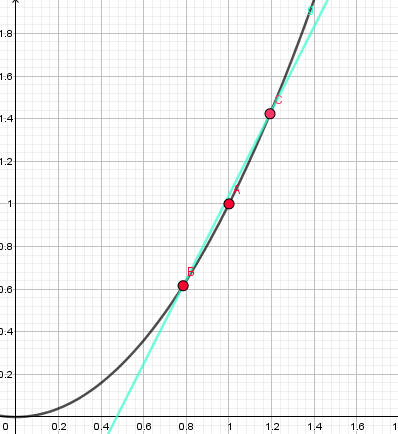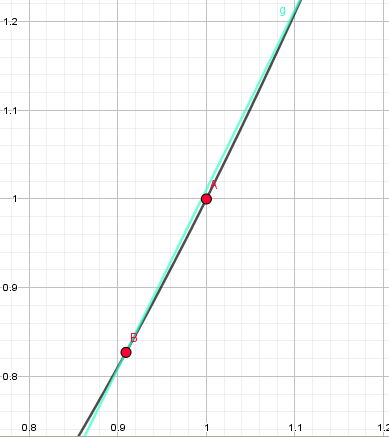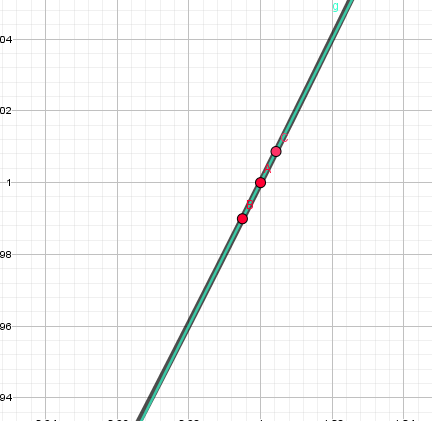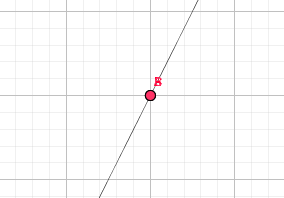(假设你已经看明白了，如果没有，你就假装看懂了 :) )

OK，那么接下来，让我们正式开始导数的学…哦不！可能还要加点料才行….

## 1.3 极限？

1000m

500m

200m

100m

1m

0.1m

0.01m

0.0000000000001m

0.0000000000000000000000000001m

OKOK，理解了，那么让我们在式子上再加点花样吧!

（顺便说一句，这里不一定必须用 $f$作为函数的标识，如果你喜欢，也可以写

## 1.4 斜率的计算### 1.4.1 小结

1. 在要求斜率的点 $(x, f(x))$附近找出一个与它很靠近的点 $(x + h, f(x + h))$
2. 把斜率用 $\frac { C点纵坐标 - A点纵坐标 } { C点横坐标 - A点横坐标 }$表示出来！
3. 在式子前面加上 $\lim_ { h\to 0 }$，代入进去计算斜率！

## 1.5 导函数

$x$对应的函数值 $y$ 是函数的值 是函数 $f(x)$的图像上，横坐标为 $x$的这一点的求导结果

(注：零点：函数的零点就是 $函数 = 0$这个方程的解。

$f(x) = ax ^ 2 + bx + c$为例。它求导的结果是 $f ‘(x)=2ax+b’$

$f ‘(x)=0’$的解就是 $x = -\frac { b } { 2a }$。这是不是与你在课堂上学到的二次函数的最值完全一致？

1. 求导，得到 $f ‘(x)=2x’$.
2. 画出 $f ‘(x)=2x’$的图像。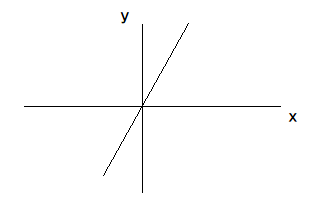3. 准备开始画图！先找出一个起点，这里我们取 $f(0)$这一点，也就是 $A(0, 2)$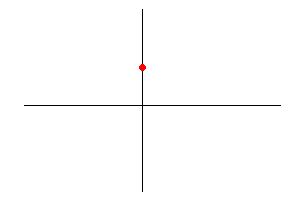4. 按照 $f ‘(x)’$的图像所示，y轴的右边应该都是上升的，而且斜率越来越大，也就是：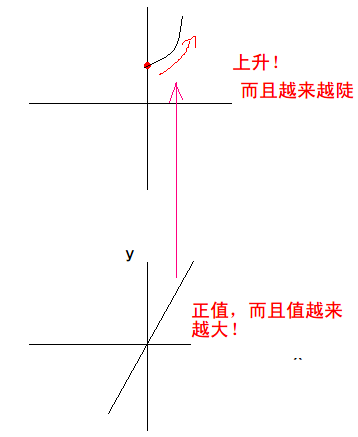5. 同理，画出左半边：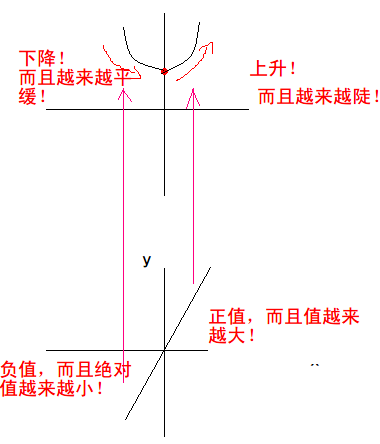### 1.5.1 导数的表示方法$a$ $b$，到底谁才是自变量？谁是常量？$y = f(x)$关于 $x$求导可以表示成：

( 正因如此，他的符号至今仍在大学数学中频频现身。)

Warning! 已经发现 LiveRe 评论系统在部分华为设备上无法加载。如果下方没有显示评论系统或评论按钮无法点击，请考虑换用其他设备浏览。